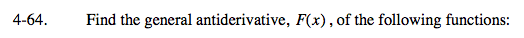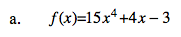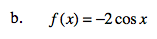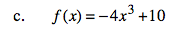### Home > CALC > Chapter 4 > Lesson 4.2.2 > Problem4-64

4-64.
1. Find the general antiderivative, F(x), of the following functions: Homework Help ✎

1. f(x) = l5x4 + 4x − 3

2. f(x) = −2cos x

3. f(x) = −4x3 + 10'Undo' the Power Rule.

3x5 + 2x2 − 3x + 3CHow does the coefficient affect the shape of the original graph? How does it affect the slope of the original graph?See (a).